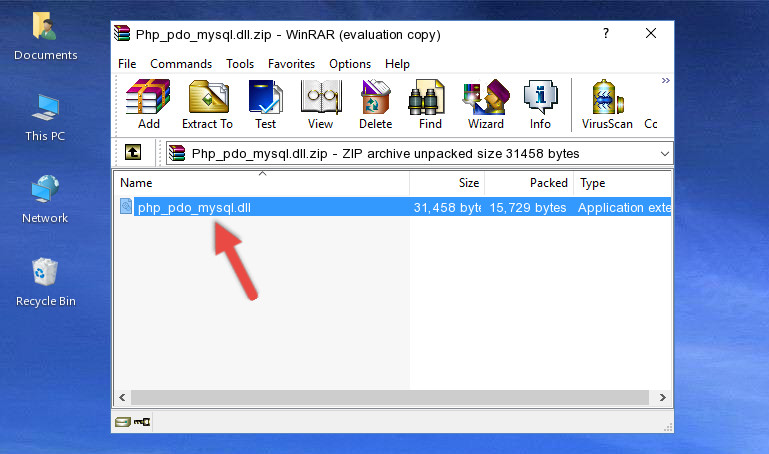# PDO实现学生管理系统_php实例

PDO配置。打开php.ini配置文件，找到下图所示的配置信息，去掉要启用的PDO前面的“#”号即可PHP pdo单例模式连接数据库'; if ( self::\$pdo ==// pdo对象的query方法返回一个PDOStatement对象 调用PDOStatement对<html> <h2>学生信息管理</h2> <a href="index.php" rel="external nofollow" >浏览学生</a> <a href="add.php" rel="external nofollow" >增加学生</a> <hr> </html>index.php<html> <head> <meta charset="UTF-8"> <title>学生信息管理系统</title> </head> <script> function doDel(id){ if(confirm("是否要删除")){ window.location='action.php?action=del&id='+id; } } </script> <body> <center> <?php include("menu.php");?> <h3>浏览学生信息</h3> <table width="600" border="1"> <tr> <th>ID</th> <th>姓名</th> <th>姓别</th> <th>年龄</th> <th>班级</th> <th>操作</th> </tr> <?php //1. 连接数据库 try{ \$pdo = new PDO("mysql:host=localhost;dbname=myapp;", "root", ""); }catch(PDOException \$e){ die("fail to connect db".\$e->getMessage()); } //2. 执行数据库，并解析遍历 \$sql = "SELECT * FROM users"; foreach(\$pdo->query(\$sql) as \$val){ echo "<tr>"; echo "<td>{\$val['id']}</td>"; echo "<td>{\$val['name']}</td>"; echo "<td>{\$val['sex']}</td>"; echo "<td>{\$val['age']}</td>"; echo "<td>{\$val['class']}</td>"; echo "<td> <a href='javascript:doDel({\$val['id']})'>删除</a> <a href='edit.php?id={\$val['id']}'>修改</a> </td>"; echo "</tr>"; } ?> </table> </center> </body> </html>

<html> <head> <meta charset="UTF-8"> <title>学生信息管理系统</title> </head> <body> <center> <?php include("menu.php");?> <h3>增加学生信息</h3> <form action="action.php?action=add" method="post"> <table> <tr> <td>姓名</td> <td><input type="text" name="name"/></td> </tr> <tr> <td>姓别</td> <td> <input type="radio" name="sex" value="m"/>男 <input type="radio" name="sex" value="w"/>女 </td> </tr> <tr> <td>年龄</td> <td><input type="text" name="age"/></td> </tr> <tr> <td>班级</td> <td><input type="text" name="class"/></td> </tr> <tr> <td> </td> <td> <input type="submit" value="增加"/> <input type="submit" value="重置"/> </td> </tr> </table> </form> </center> </body> </html>

edit.php

<html> <head> <meta charset="UTF-8"> <title>学生信息管理系统</title> </head> <body> <center> <?php include("menu.php"); //获取修改信息 //1. 连接数据库 try{ \$pdo = new PDO("mysql:host=localhost;dbname=myapp;", "root", ""); }catch(PDOException \$e){ die("fail to connect db".\$e->getMessage()); } //2. 拼装sql语句，取出信息 \$sql = "SELECT * FROM users WHERE id=".\$_GET['id']; \$stmt = \$pdo->query(\$sql); if(\$stmt->rowCount() > 0){ \$stu = \$stmt->fetch(PDO::FETCH_ASSOC); //解析数据 }else{ die("没有修改的信息"); } ?> <h3>修改学生信息</h3> <form action="action.php?action=edit" method="post"> <!-- 以隐藏域的方式添加id --> <input type="hidden" name="id" value="<?php echo \$stu['id']; ?>"> <table> <tr> <td>姓名</td> <td><input type="text" name="name" value="<?php echo \$stu['name'];?>"/></td> </tr> <tr> <td>姓别</td> <td> <input type="radio" name="sex" value="m" <?php echo (\$stu['sex']== "m")? "checked": ""; ?>/>男 <input type="radio" name="sex" value="w" <?php echo (\$stu['sex']== "w")? "checked": ""; ?>/>女 </td> </tr> <tr> <td>年龄</td> <td><input type="text" name="age" value="<?php echo \$stu['age'];?>"/></td> </tr> <tr> <td>班级</td> <td><input type="text" name="class" value="<?php echo \$stu['class'];?>"/></td> </tr> <tr> <td> </td> <td> <input type="submit" value="修改"/> <input type="submit" value="重置"/> </td> </tr> </table> </form> </center> </body> </html>

action.php

<?php //1. 连接数据库 try{ \$pdo = new PDO("mysql:host=localhost;dbname=myapp;", "root", ""); }catch(PDOException \$e){ die("fail to connect db".\$e->getMessage()); } //2. 通过action的值做相应的操作 switch(\$_GET['action']){ case "add": //增加操作 \$name = \$_POST['name']; \$sex = \$_POST['sex']; \$age = \$_POST['age']; \$class = \$_POST['class']; \$sql = "INSERT INTO users VALUES (null, '{\$name}','{\$sex}', '{\$age}', '{\$class}')"; \$rw = \$pdo->exec(\$sql); if(\$rw > 0){ echo "<script>alert('增加成功'); window.location='index.php'</script>"; }else{ echo "<script>alert('增加失败'); window.history.back()</script>"; } break; case "del": \$id = \$_GET['id']; \$sql = "DELETE FROM users WHERE id={\$id}"; \$pdo->exec(\$sql); header("location:index.php"); break; case "edit": \$name = \$_POST['name']; \$sex = \$_POST['sex']; \$age = \$_POST['age']; \$class = \$_POST['class']; \$id = \$_POST['id']; \$sql = "UPDATE users SET name='{\$name}',sex='{\$sex}',age={\$age},class={\$class} WHERE id={\$id}"; \$rw = \$pdo->exec(\$sql); if(\$rw > 0){ echo "<script>alert('修改成功'); window.location='index.php'</script>"; }else{ echo "<script>alert('修改失败'); window.history.back()</script>"; } break; }

Php中的pdo是什么意思啊？举个例子指点迷津？？！

pdo就是把操作数据库的函数封装成一个pdo类，其间做了安全验证而已。

<!--?php

define('DB_NAME', 'pdo_test');

define('DB_USER', 'root');

define('DB_HOST', 'localhost');

define('DB_CHARSET', 'utf8');

try {

\$DBH = new PDO('mysql:host='.DB_HOST.';dbname='.DB_NAME, DB_USER, DB_PASSWORD);

\$DBH--->exec('SET CHARACTER SET '.DB_CHARSET);

\$DBH->exec('SET NAMES '.DB_CHARSET);

} catch (PDOException \$e) {

print "Error!: " . \$e->getMessage() . "<br>";

die();

}

?>

<!--?php

//增加数据

\$sql_insert = 'Insert INTO wp_options(blog_id,option_name,option_value,autoload) VALUES (0,'.time().rand(1,100).','.time().rand(1,100).',\'no\')';

\$back = \$DBH--->exec(\$sql_insert); //返回 bool 的true or fal

\$lastInsertId = \$DBH->lastInsertId();

//删除数据

\$sql_delete = 'Delete FROM wp_options Where option_id='.\$lastInsertId;

\$back = \$DBH->exec(\$sql_delete); //返回 bool 的true or fal

\$lastInsertId = \$DBH->lastInsertId();

//更新数据

\$sql_update = 'Update wp_options SET option_name = \''.time().rand(1,100).'\' Where option_id='.\$lastInsertId;

\$lastUpdateId = \$DBH->lastInsertId(); //返回的对应的操作的id

//查询数据

\$sql_select = 'Select option_id FROM wp_options orDER BY option_id DESC LIMIT 4 ';

\$back = \$DBH->query(\$sql_select); //返回一个对象 这个对象可以用foreach 直接遍历循环 循环的为查询的结果集

\$back = \$DBH->query(\$sql_select)->fetch(); //返回一条数据结果 这个对象可以用foreach 直接遍历循环 循环的为查询的结果集

\$back = \$DBH->query(\$sql_select)->fetchAll(); //返回一个数组 这个对象可以用foreach 直接遍历循环 循环的为查询的结果集

\$back = \$DBH->query(\$sql_select)->fetchColumn(0); //返回一个字段字符串，这个字符串是返回的记录的第一条记录的第一个字段

?>

php mysql pdo 操作类 怎么实例化

new PDO(\$dsn, \$user, \$pass, \$driver_options);

php 使用pdo对mysql数据库增删改查实例

//连接、查询

try {

\$dbh = new PDO('mysql:host=localhost;dbname=test', \$user, \$pass);

foreach(\$dbh->query('SELECT * from FOO') as \$row) {

print_r(\$row);

}

\$dbh = null;//释放资源

} catch (PDOException \$e) {

print "错误!: " . \$e->getMessage() . "<br></br>";

die();

}

//执行删除操作，插入、修改与此类似，更改SQL语句即可

\$count = \$dbh->exec("DELETE FROM fruit WHERE colour = 'red'");

php中如何在自定义的类中调用pdo中的方法

\$tt=new test();

\$tt->pdo();追问不是这样，

class A{

}

function pod(){

\$pdo->prepare(\$sql);

}

• 本文相关：
• php实现学生管理系统
• thinkphp访问不存在的模块跳转到404页面的方法
• php利用事务处理转账问题
• php使用gifencoder类生成的gif动态图片验证码
• zend framework基于command命令行建立zf项目的方法
• swoole-1.7.22 版本已发布，修复php7相关问题
• php防止表单重复提交实例讲解
• 我整理的php 7.0主要新特性
• thinkphp3.x连接mysql数据库的方法(具体操作步骤)
• 怎样给php源代码加密?php二进制加密与解密的解决办法
• laravel 5.1下php artisan migrate的使用注意事项总结
• Php中的pdo是什么意思啊？举个例子指点迷津？？！
• php mysql pdo 操作类 怎么实例化
• php 使用pdo对mysql数据库增删改查实例
• php中如何在自定义的类中调用pdo中的方法
• 如何在PHP下开启PDO MySQL的扩展
• PHP中用PDO向Mysql里输入数据
• PHP中，实例化pdo对象以后PDOStatement类就能用了吗？
• 为什么 PHP 应该使用 PDO 方式访问数据库
• php pdo单例模式怎么同时连接两个数据库
• PHP使用PDO连接Mysql的返回值问题
• 免责声明 - 关于我们 - 联系我们 - 广告联系 - 友情链接 - 帮助中心 - 频道导航